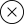# Welcome to Math for Elementary Teachers

In this course, you will explore math for elementary teachers. You will start by looking at cardinality, and then will move on to examine place value. From there, you will examine the number system, and also operations and integers. You will also learn about fraction concepts and computation with fractions, as well as measurement. You will examine perimeter and area, surface area and volume, two- and three-dimensional shapes, and plotting on the coordinate grid. You will also explore ratios and proportional relationships, as well as algebraic expressions and equations. Finally, you will take a closer look at functions, and statistics and probability.

## Learning Objectives/

#### 1. Cardinality

1. 1.1 Describe the meaning of one-to-one correspondence and how it is used when counting a group of elements.
2. 1.2 Illustrate multiple approaches for teaching the concept of counting on to add.

#### 2. Place Value

1. 2.1 Explain how multiple models can be used to teach place value.
2. 2.2 Explain how to represent a number in multiple formats.

#### 3. The Number System

1. 3.1 Illustrate how sets of numbers within the real number system are related using a visual model, such as a Venn diagram.
2. 3.2 Sort real numbers into categories of numbers.

#### 4. Operations on Integers

1. 4.1 Illustrate ways to model groups of integers, including negative integers.
2. 4.2 Describe various ways to teach the concept of adding and subtracting integers.
3. 4.3 Describe various ways to teach the concept of multiplying and dividing integers.
4. 4.4 Describe how to evaluate numerical expressions according to the order of operations.

#### 5. Fraction Concepts and Computation with Fractions

1. 5.1 Describe multiple ways to teach the concept of a fraction to students.
2. 5.2 Explain different approaches for adding, subtracting, multiplying, and dividing fractions, including the use of models.

#### 6. Measurement

1. 6.1 Identify metric measurements.
2. 6.3 Identify customary measurements.
3. 6.4 Describe ways to convert between, and within, the metric and customary systems and provide real-world problems that utilize such conversions.

#### 7. Perimeter and Area

1. 7.1 Explain ways to teach and use the concept of perimeter.
2. 7.2 Explain ways to teach and use the concept of perimeter.
3. 7.3 Explain the relationship between area and perimeter and the effect of scaling on the relationships between measurements of area and perimeter.

#### 8. Surface Area and Volume

1. 8.1 Explain ways to teach and use the concept of surface area.
2. 8.2 Explain ways to teach and use the concept of volume.
3. 8.3 Explain the relationship between surface area and volume and the effect of scaling on the relationships between the measurements of surface area and volume.

#### 9. Two- and Three-Dimensional Shapes

1. 9.1 Describe attributes of two- and three-dimensional shapes.
2. 9.2 Illustrate how to sort shapes, according to their attributes.
3. 9.3 Draw nets of different three-dimensional shapes.

#### 10. Plotting on the Coordinate Grid

1. 10.1 Describe ways to teach the components of a coordinate grid and how to plot points in all four quadrants.
2. 10.2 Describe different real-world problems that may be solved using plotting of points on a coordinate grid.

#### 11. Ratios and Proportional Relationships

1. 11.1 Describe the concept of a ratio and how to solve real-world problems involving ratios.
2. 11.2 Describe the concept of a proportion and how to solve real-world problems involving proportions.
3. 11.3 Explain how proportional relationships are connected to linear relationships.

#### 12. Algebraic Expressions and Equations

1. 12.1 Illustrate how to evaluate different algebraic expressions.
2. 12.2 Illustrate multiple ways for teaching the process of solving equations to students, including the use of a balance scale.
3. 12.3 Provide examples of real-world problems that may be solved by writing, and evaluating/solving, algebraic expressions/equations.

#### 13. Functions

1. 13.1 Explain ways to illustrate the concept of a function to students.
2. 13.2 Describe how to evaluate a function for different input values and connect to tabular and graphical displays.
3. 13.3 Compare features of functions using multiple representations, including graphs and equations.

#### 14. Statistics and Probability

1. 14.1 Explain ways to teach simple and compound probability, as well as the counting principle.
2. 14.2 Explain patterns of bivariate data using scatter plots and two-way frequency tables.

There are no reviews yet.

## RELATED COURSES